Next: Maple Worksheets Reference Up: Using Maple Efficiently Previous: Building Complicated Maple

## Common problems

This section contains a list of common Maple problems and their solutions. You are also free to get help from your instructor or any of the class helpers. The problems are listed in a rough order of severity, from most to least. If you don't find your problem here, or the suggestions don't work for you, ask for help!

1. Maple just crashed
This happens from time to time. It may be caused by a bug in the program, or by something you have done that has totally confused Maple. The biggest danger is that you will lose your work. The best defence is to save your worksheet often, using the Save item on the File menu. Saving your work often can save you a tremendous amount of time.

2. Missing semicolon
Suppose you forget to put a semicolon at the end of a Maple command, as in the following example. Notice that the syntax error appears after the next command.

```  > p := x^2+3
```

```  > q := x^12;
```

```syntax error:
q := x^12;
^

```

The fix is to go back to the first line and add the semicolon. This usually does the trick. If it doesn't and you get another syntax error, try issuing the first command again. If this doesn't solve the problem, you might want to ask for help.

3. Assigning and unassigning values to variables
Suppose you set a variable x to have the value 2, but later want to use x as a variable again. The trick is to use the command x := 'x'; as shown below.

```  > x := 2;
``````  > f := x -> x^2;
```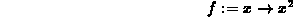```  > f(x);
```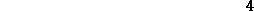```  > diff(f(x),x);
```

```Error, wrong number (or type) of parameters in function diff

```

```  > x := 'x';
``````  > diff(f(x),x);
```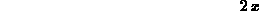4. Value not assigned
Suppose you forget the semicolon (:) in an assignment statement. Then Maple will treat this as an equation and not an assignment. See the following example.

```  > f := x -> x^2;
``````  > a := 2;
``````  > b = 2;
```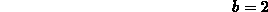```  > f(a);
``````  > f(b);
```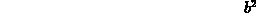5. Empty plot warnings Sometimes Maple gives you the error message Warning in iris-plot: empty plot and refuses to do the plot. The usual reason for this error is that Maple can't compute numerical values of the expression you asked it to plot. This can be caused by a your expression depending on extra parameters or missing * for multiplication or other syntax error in your expression. It can also happen if you try to use square brackets, [], or curly braces, {}, in expressions to indicate association. You can only use parentheses, () for this purpose. Maple use square brackets to indicate a list and curly braces to indicate a set.

Some examples are shown below.

```  > q := x^2+xy-3;
```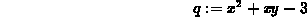```  > plot3d(q,x=0..1,y=0..1);
```

```Warning in iris-plot: empty plot

```

```  > p := [sqrt(x)-1]^3;
```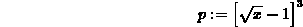```  > plot(p,x=0..1);
```

```Warning in iris-plot: empty plot

```

6. Problems with the seq command
The Maple seq command has the undesirable side-effect shown in the example below.

```  > seq(i^2,i=1..5);
``````  > i;
```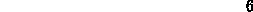The side-effect is that the index variable i ends up having the value 6 after the seq command is executed. This is not a problem unless you want to use i as a variable later on in your Maple session, as shown in the example below. The last two commands in the example show how to solve this problem.

```  > f := i -> i^3;
```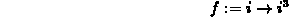```  > f(i);
```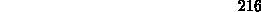```  > i := 'i';
```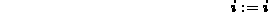```  > f(i);
```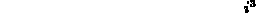7. Too many page breaks
This is a problem with a printed worksheet having too many page breaks. The most likely cause is that your worksheet has double separator lines. By default, a double separator line in a Maple worksheet causes a page break at that point.

To fix this problem, open up your worksheet and delete any extra separator lines. To delete a separator line, click on it with the leftmost mouse button. (A very small black square will appear at the left end of the separator line when you've selected the line correctly. Make sure that this black square has appeared before going on to the next step.) Select the Delete Cursor Region item from the Edit menu, and the separator line should disappear. You can also use the keyboard shortcut Ctrl+Del, i.e. pressing the Ctrl key and the Delete key at the same time. Save your worksheet to make the change effective before exiting Maple.

8. Quota problems
Each user is allowed a certain amount, called a quota, of storage space in their home directory. If you get a message saying you are over quota when you log in, then you must delete some files. The danger here is that you may not be able to save files until you are back under quota.

Here are some things to watch out for.

• Keeping Maple plot files around after you've turned in the lab. You should delete these files with the rm command after you have printed them out.
• Pasting plots into your Maple worksheet. Avoid doing this. The plots take up much more space when they are pasted into a worksheet than they do saved as a separate file.
Suppose you had a file called junkfile in your home directory. Then the command to delete this file is the following.
```% rm junkfile
```
To remove all files of a certain kind, you can use the Unix wildcard matching character *. For example, the command
```rm *.ps
```
would remove all files ending with .ps from your directory.Next: Maple Worksheets Reference Up: Using Maple Efficiently Previous: Building Complicated Maple

William W. Farr
Mon Aug 28 09:31:56 EDT 1995